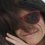# A Proof(??) of harmonic series being divergent

$$1 + \frac{1}{2} + \frac{1}{3} + \frac{1}{4} + ... =$$

$(1 + \frac{1}{2} + \frac{1}{4} + ... \frac{1}{2^n})(1 + \frac{1}{3} + \frac{1}{9} + ... \frac{1}{3^n}) ... (1 + \frac{1}{p} + \frac{1}{p^2} + ... \frac{1}{p^n})$

Where $'p'$ is a prime number.

As each of the sums in the brackets are >1, the product of all of these terms is divergent (as there are infinitely many primes and the product of infinite terms all >1 can't be finite*). Therefore, the harmonic series is divergent.

I decided to write this after reading other proofs of this. It's most likely already been done more rigorously centuries ago so if you know who first came up with a similar proof please post a link in the comment section. And also if anyone could actually prove point *. Sorry if the title ('proof') was misleading.Note by Nucky Korprasertsri
7 years, 5 months ago

This discussion board is a place to discuss our Daily Challenges and the math and science related to those challenges. Explanations are more than just a solution — they should explain the steps and thinking strategies that you used to obtain the solution. Comments should further the discussion of math and science.

When posting on Brilliant:

• Use the emojis to react to an explanation, whether you're congratulating a job well done , or just really confused .
• Ask specific questions about the challenge or the steps in somebody's explanation. Well-posed questions can add a lot to the discussion, but posting "I don't understand!" doesn't help anyone.
• Try to contribute something new to the discussion, whether it is an extension, generalization or other idea related to the challenge.

MarkdownAppears as
*italics* or _italics_ italics
**bold** or __bold__ bold
- bulleted- list
• bulleted
• list
1. numbered2. list
1. numbered
2. list
Note: you must add a full line of space before and after lists for them to show up correctly
paragraph 1paragraph 2

paragraph 1

paragraph 2

[example link](https://brilliant.org)example link
> This is a quote
This is a quote
    # I indented these lines
# 4 spaces, and now they show
# up as a code block.

print "hello world"
# I indented these lines
# 4 spaces, and now they show
# up as a code block.

print "hello world"
MathAppears as
Remember to wrap math in $$ ... $$ or $ ... $ to ensure proper formatting.
2 \times 3 $2 \times 3$
2^{34} $2^{34}$
a_{i-1} $a_{i-1}$
\frac{2}{3} $\frac{2}{3}$
\sqrt{2} $\sqrt{2}$
\sum_{i=1}^3 $\sum_{i=1}^3$
\sin \theta $\sin \theta$
\boxed{123} $\boxed{123}$

Sort by:

You can also group these terms as such : 1 + (1/2) + (1/3+1/4) + ( 1/5+1/6+1/7)+( 1/8+1/9+1/10+1/11+1/12) and so forth... => 1 + 1/2+ 7/12 + 107/210 +2021/3960...

7/12>1/2 , 107/210>1/2 and 2021/3960 > 1/2. Thus, this series diverges slowly.

- 6 years, 10 months ago

Hi Nucky,

The idea an infinite product of terms all greater than 1 implies that the series diverges turns out not to be true. A famous example is $\prod_{k=1}^{\infty}(1 + \frac{1}{a_k}) = e$ where $a_1 = 1$ and $a_{k+1} = (k+1)(a_k +1)$ for all $k > 1.$ I tried proving the harmonic series diverges in a similar way once and had the same assumption!

- 6 years, 5 months ago